# Project Euler – Problem 66 Solution

Check out my new course Learn you some Lambda best practice for great good! and learn the best practices for performance, cost, security, resilience, observability and scalability.

#### Problem

Consider quadratic Diophantine equations of the form:

x2 – Dy2 = 1

For example, when D=13, the minimal solution in x is 6492 – 13×1802 = 1.

It can be assumed that there are no solutions in positive integers when D is square.

By finding minimal solutions in x for D = {2, 3, 5, 6, 7}, we obtain the following:

32 – 2×22 = 1

22 – 3×12 = 1

92 – 5×42 = 1

52 – 6×22 = 1

82 – 7×32 = 1

Hence, by considering minimal solutions in x for D <= 7, the largest x is obtained when D=5.

Find the value of D <= 1000 in minimal solutions of x for which the largest value of x is obtained.

#### Solution

```// define function to get the continued fractions, a0,a1,a2,...an
let continuedFraction D =
// define functions for working out the nth term of P, Q and a sequence
let getPn an' qn' pn' = an' * qn' - pn'
let getQn D pn qn' = (D - pown pn 2) / qn'
let getAn a0 pn qn = (a0 + pn) / qn

// work out the initial terms
let a0 = bigint(sqrt(double(D)))
let p1, q1 = a0, D-a0*a0
let a1 = getAn a0 p1 q1
let initial = (p1, q1, a1)

Seq.unfold (fun (pn', qn', an') ->
let pn = getPn an' qn' pn'
let qn = getQn D pn qn'
let an = getAn a0 pn qn
Some((pn', qn', an'), (pn, qn, an))) initial
|> Seq.map (fun (pn, qn, an) -> an)
|> Seq.append [a0]

// define function to get the continued fraction convergents
// e.g. for D = 7: 2/1, 3/1, 5/2, 8/3, ...
let continuedFractionConvergents D =
let getN an n' n'' = an * n' + n''

// work out the initial terms
let fractions = continuedFraction D
let p0, p1 = a0, a0 * (Seq.nth 1 fractions) + 1I
let q0, q1 = 1I, Seq.nth 1 fractions
let initial = (p1, q1, p0, q0)

Seq.scan (fun (pn', qn', pn'', qn'') an ->
let pn = getN an pn' pn''
let qn = getN an qn' qn''
(pn, qn, pn', qn')) initial (fractions |> Seq.skip 2)
|> Seq.map (fun (pn, qn, pn', qn') -> (pn, qn))
|> Seq.append [(p0, q0)]

[1I..1000I]
|> List.filter (fun d -> sqrt(double(d)) % 1.0 <> 0.0)
|> List.maxBy (fun d ->
continuedFractionConvergents d
|> Seq.filter (fun (x, y) -> x*x - d*y*y = 1I)
|> Seq.map fst
```

After spending much time reading the information on Pell’s equation on mathworld and wikipedia, it turns out that the best way to solve the equation for a given D is to work out the continued fraction convergents to the square root of D.

For example, for D = 7, the equation becomes: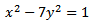The fundamental solution (the first pair of x, y which satisfies the equation) can be obtained by going through the sequence of convergents for the square root of 7: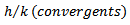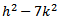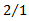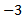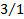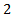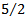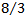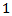So how do we workout the values of the convergents? The continued fraction for a number is usually written as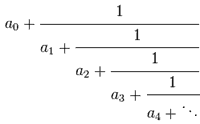The first ten values generated by the continued fraction for the square root of 2 are:

1, 3/2, 7/5, 17/12, 41/29, 99/70, 239/169, 577/408, 1393/985, 3363/2378, …

These values are called continued fraction convergents, getting closer to the true value of square root 2 with each step..

So, to find the convergents to the square root of D, we first need to find the values of a: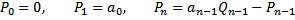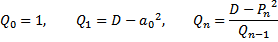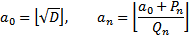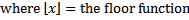Once we have the sequence of values for a, we can then find out the convergent values: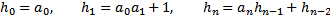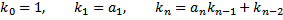The rest is simple, for each value of D between 1 and 1000, go through the convergents to find the fundamental solution and return the max value of x in all the fundamental solutions.Hi, I’m Yan. I’m an AWS Serverless Hero and the author of Production-Ready Serverless.

I specialise in rapidly transitioning teams to serverless and building production-ready services on AWS.

Are you struggling with serverless or need guidance on best practices? Do you want someone to review your architecture and help you avoid costly mistakes down the line? Whatever the case, I’m here to help.Check out my new course, Learn you some Lambda best practice for great good! In this course, you will learn best practices for working with AWS Lambda in terms of performance, cost, security, scalability, resilience and observability. Enrol now and enjoy a special preorder price of £9.99 (~\$13).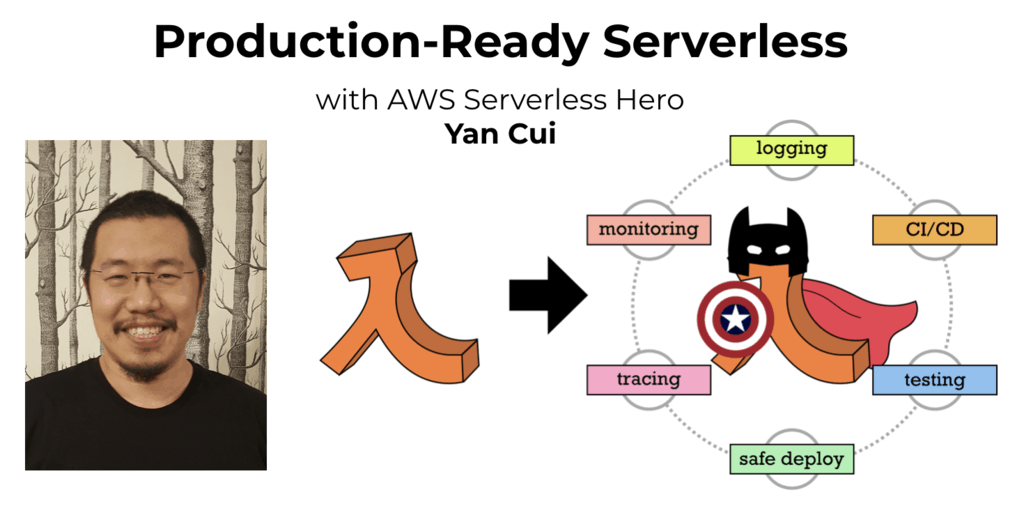Are you working with Serverless and looking for expert training to level-up your skills? Or are you looking for a solid foundation to start from? Look no further, register for my Production-Ready Serverless workshop to learn how to build production-grade Serverless applications!